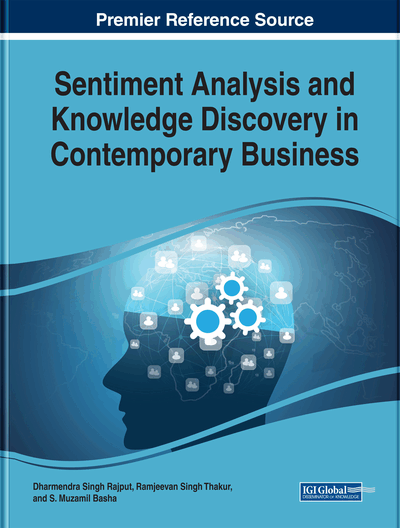# Introduction and Implementation of Machine Learning Algorithms in R

S. R. Mani Sekhar (M. S. Ramaiah Institute of Technology, India) and G. M. Siddesh (M. S. Ramaiah Institute of Technology, India)
DOI: 10.4018/978-1-5225-4999-4.ch008
Available
\$37.50
No Current Special Offers

## Abstract

Machine learning is one of the important areas in the field of computer science. It helps to provide an optimized solution for the real-world problems by using past knowledge or previous experience data. There are different types of machine learning algorithms present in computer science. This chapter provides the overview of some selected machine learning algorithms such as linear regression, linear discriminant analysis, support vector machine, naive Bayes classifier, neural networks, and decision trees. Each of these methods is illustrated in detail with an example and R code, which in turn assists the reader to generate their own solutions for the given problems.
Chapter Preview
Top

## Linear Regression

Linear regression (Kenney & Keeping, 1962) is a Machine learning method that is used to model the relationship between the two variables one of which is called explanatory variable or independent variable and the other one is called target variable or dependent variable.

If there is only one explanatory variable then the problem is called simple linear regression whereas if there are more explanatory variable then the problem is called multiple linear regression.

Mathematically, the relationship modeled using linear regression approach yields a straight line when plotted as a graph. Conventionally, the explanatory variable is represented by X and target variable is represented by Y.

The general mathematical equation for linear regression (Kenney & Keeping, 1962) is given by,

Y = AX + B ; where A & B are constants.(1)

Linear regression is used when there is a some set of known pairs of X and Y values and we have to predict new Y value for every new X value using the predefined model as shown in equation 1. The widely used approach to fit the linear regression model is “Least Square Method” (Zhou & Han, 1951). Linear regression approach is used whenever there is a task of modeling or analysis of several variables.

The real life example of the linear regression is predicting the weight of the person whose height is known or else predicting the distance that the car will travel whose speed is known or predicting the weather where temperature is known.

Consider the example for predict the weight of the person whose height is known. For this, first collect the sample data where the heights and weights of the person are mentioned. Using the collected data, generate a relationship model by applying linear regression. Simultaneously find out the value of the constants from the model created. Thereafter plot the graph and obtain the line which is always straight. Now, the model can be used to predict the weight for the given person height. Table 1, show the sample dataset named “trees”(Ryan, Joiner, & Ryan,1976) it is a build-in dataset of RStudio consist of 4 columns, which represent the Girth, Height and volume of trees.

Table 1.
Sample dataset of trees
 Girth Height Volume 1 8.3 70 10.3 2 8.6 65 10.3 3 8.8 63 10.2 4 10.5 72 16.4

(Ryan, Joiner, & Ryan, 1976)

## Key Terms in this Chapter

Machine Learning: Helps machine to perform action without being explicitly coding.

Data Preprocessing: Converting raw data into valuable form.

Predict: Helps in forecasting undefined event.

Algorithm: Sequence of instructions helps in performing certain computation.

Classifier: Helps in grouping of input data to a category.

Dataset: Collection of data.

Feature: A measurable property which helps in prediction of necessary information.

## Complete Chapter List

Search this Book:
Reset